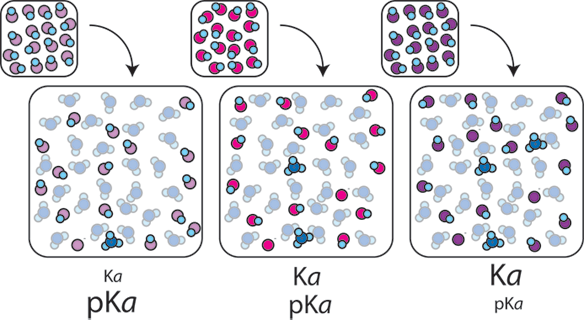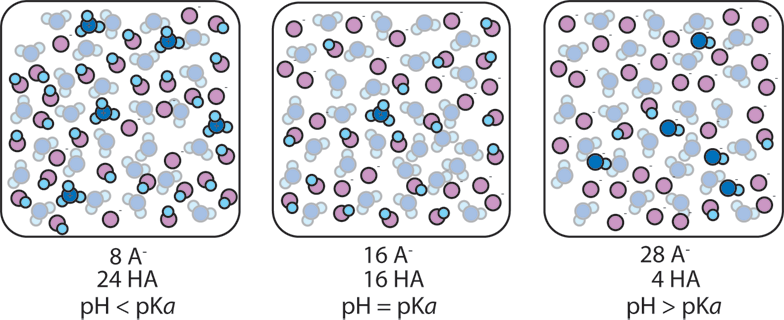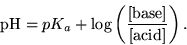# How can we predict the pH of a buffer?

### Predicting the pH of a Buffer

The pH of a buffer is determined by two factors; 1) The equilibrium constant Ka of the weak acid and 2) the ratio of weak base [A-] to weak acid [HA] in solution.

1) Different weak acids have different equilibrium constants (Ka). Ka tells us what proportion of HA will be dissociated into H+ and A- in solution. The more H+ ions that are created, the more acidic and lower the pH of the resulting solution.2) The ratio of A- to HA in a buffer also affects the pH. If a buffer has more base than acid, more OH- ions are likely to be present and the pH will rise. If a buffer has more acid than base, more H+ ions are present and the pH will fall. When the concentrations of A- and HA are equal, the concentration H+ is equal to Ka, (or equivalently pH = pKa).This is formalized in the Henderson-Hasselbalch equation:Since pKa is a property of the weak acid we select for our buffer, we can control the pH by manipulating the proportion of weak base (A-) and weak acid (HA) in solution.

The content above has been converted from Adobe Flash Player and may not display correctly.

When [A-]/[HA] remains close to 1, the pH remains close to pKa. As [A-]/[HA] goes from 1/10 to 10 the pH changes from pKa+1 to pKa-1. So as long as [A-]/[HA] is between 1/10 and 10, the pH is within 1 unit of pKa. The addition of a strong acid or base to a buffer changes the ratio [A-]/[HA]. When a buffer absorbs an acid (H+), the acid converts A- to HA (A- + H+ → HA) and so the ratio [A-]/[HA] decreases. When a buffer absorbs base (OH-), the base converts HA to A- (HA + OH- → A-) and so the ratio [A-]/[HA] increases. As long as the added acid or base doesn't cause [A-]/[HA] to go outside the range 1/10..10, the pH will remain within 1 unit of pKa, i.e. the pH won't change by very much and the solution is therefore buffered.

See the next two pages for practice in determining the pH of a buffer solution.